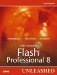# Running the Function

 Now that you have your function, let's make it work for you. To run this function (also called invoking or calling the function), you start with your function name, followed by a set of parentheses to hold your parameters, and you finish with a semicolon to end the line, as shown here: ` function myFunction ():Void{ trace ("My first function"); } myFunction(); //output: My first function ` That was easy. You created a function and then invoked it to see the statements run, and the message displayed in the Output panel. Let's now look at another way of creating functions. This way starts with the function name that we set to the Function type. Then you set it equal to the keyword function, followed by a set of parentheses, a colon, and the return value type. Then the curly brackets for holding your code are added. Here's an example using a generic template: ` var myFunction:Function = function():ReturnType{ //script to run in function } ` Now let's put this into practice with the previous example: ` var myFunction:Function = function():Void{ trace("My second function"); } myFunction(); //output: My second function ` Now you have seen two basic ways of creating functions and running them. So far, all you have done is run a simple trace statement. Next, let's put some script in it that you can really use. We'll start with a function that fades out a movie: ` function fadeOut():Void{ myMovie_mc._alpha -= 5; } //now invoke the function fadeOut(); ` Every time this function is invoked, the movie clip myMovie_mc will decrease its alpha value by 5. You would normally place this function within a looping event such as an onEnterFrame event. (For more on events, see Chapter 14.) That was simple, but what if you only wanted to fade to a certain point? You can place conditional as well as loop statements within functions to perform a conditional test. Here's the same example, but this time we're using an if statement to only allow myMovie_mc to fade to a certain point: ` function fadeOut():Void{ if(myMovie_mc._alpha >50){ myMovie_mc._alpha-=5; } } //now invoke the function fadeOut(); ` Now the function will check whether the movie clip has faded to the designated point yet. If it has reached the designated point, the function still runs, but the code in the if statement will not. Suppose you want to set the point where the alpha will fade differently for two different functions that are invoked. This is where parameters come in.Macromedia Flash Professional 8 Unleashed
ISBN: 0672327619
EAN: 2147483647
Year: 2005
Pages: 319

flylib.com © 2008-2017.
If you may any questions please contact us: flylib@qtcs.net